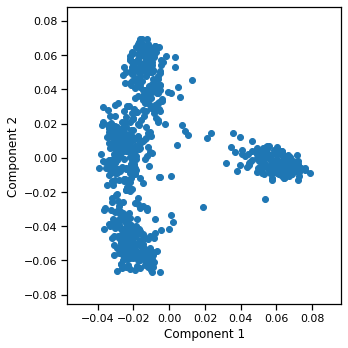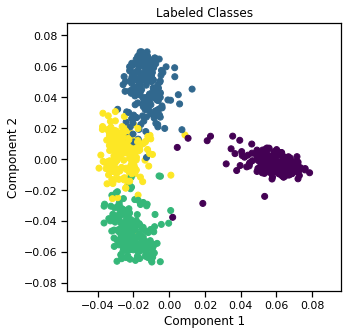# Using quick_visualize() to quickly understand multi-view data¶

## Easily view and understand underlying clusters in multi-view data¶

As a simple example, say we had high-dimensional multi-view data that we wanted to quickly visualize before we begin our analysis. With quick_visualize, we can easily do this. As an example, we will visualize the UCI Multiple Features dataset.

:

# Import the function
from mvlearn.plotting import quick_visualize

import matplotlib.pyplot as plt
%matplotlib inline

:

# Load 4-class data

:

# Quickly visualize the data
quick_visualize(Xs, figsize=(5,5))### If we have class labels that we want to visualize too, we can easily add those¶

:

quick_visualize(Xs, labels=y, title='Labeled Classes', figsize=(5,5))